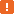# Getting the Focused Row, Column and Cell in Microsoft DataGridWhen testing an application that uses Microsoft DataGrid .NET controls, you may need to know which row, column and cell are currently focused. The DataGrid control has special internal properties that let you determine the currently selected element:

• `CurrentRowIndex` - Returns the index (zero-based) of the selected row.
• `CurrentColumn` - Returns the index (zero-based) of the currently selected column.

To determine the value in the currently selected cell, you can use the `MicrosoftDataGrid.wValue` property and pass the current row and column indexes as parameters.In order for TestComplete to be able to access internal properties of the DataGrid control, the .NET Application Support plugin must be installed and enabled. Properties and methods of the `MicrosoftDataGrid` object are only available if the Microsoft Control Support plugin is installed and enabled.

The following example demonstrates how you can use the mentioned properties to get the value in the focused cell. For more information on obtaining DataGrid cell values, see Obtaining and Setting Cell Values in Microsoft DataGrid.

JavaScript, JScript

function Main ()
{
var p, Grid, RowIndex, ColIndex;
// Obtain the grid object
p = Sys.Process("DataGridSample");
Grid = p.WinFormsObject("Form1").WinFormsObject("dataGrid1");

// Get information about the selected cell
RowIndex = Grid.CurrentRowIndex;
ColIndex = Grid.CurrentColumn;
Log.Message ("Current row: " + RowIndex);
Log.Message ("Current column: " + Grid.wColumn(ColIndex));
Log.Message ("Current cell value: " + Grid.wValue(RowIndex, ColIndex).ToString().OleValue);
}

Python

``````def Main ():
# Obtain the grid object
p = Sys.Process("DataGridSample")
Grid = p.WinFormsObject("Form1").WinFormsObject("dataGrid1")

# Get information about the selected cell
RowIndex = Grid.CurrentRowIndex
ColIndex = Grid.CurrentColumn
Log.Message ("Current row: " + RowIndex)
Log.Message ("Current column: " + Grid.wColumn(ColIndex))
Log.Message ("Current cell value: " + Grid.wValue[RowIndex, ColIndex].ToString().OleValue)``````

VBScript

Sub Main
Dim p, Grid, RowIndex, ColIndex
' Obtain the grid object
Set p = Sys.Process("DataGridSample")
Set Grid = p.WinFormsObject("Form1").WinFormsObject("dataGrid1")

' Get information about the selected cell
RowIndex = Grid.CurrentRowIndex
ColIndex = Grid.CurrentColumn
Log.Message ("Current row: " & RowIndex)
Log.Message ("Current column: " & Grid.wColumn(ColIndex))
Log.Message ("Current cell value: " & Grid.wValue(RowIndex, ColIndex).ToString.OleValue)
End Sub

DelphiScript

procedure Main;
var p, Grid, RowIndex, ColIndex : OleVariant;
begin
// Obtain the grid object
p := Sys.Process('DataGridSample');
Grid := p.WinFormsObject('Form1').WinFormsObject('dataGrid1');

// Get information about the selected cell
RowIndex := Grid.CurrentRowIndex;
ColIndex := Grid.CurrentColumn;
Log.Message ('Current row: ' + aqConvert.VarToStr(RowIndex));
Log.Message ('Current column: ' + Grid.wColumn[ColIndex]);
Log.Message ('Current cell value: ' + Grid.wValue[RowIndex, ColIndex].ToString.OleValue);
end;

C++Script, C#Script

function Main ()
{
var p, Grid, RowIndex, ColIndex;
// Obtain the grid object
p = Sys["Process"]("DataGridSample");
Grid = p["WinFormsObject"]("Form1")["WinFormsObject"]("dataGrid1");

// Get information about the selected cell
RowIndex = Grid["CurrentRowIndex"];
ColIndex = Grid["CurrentColumn"];
Log["Message"]("Current row: " + RowIndex);
Log["Message"]("Current column: " + Grid["wColumn"](ColIndex));
Log["Message"]("Current cell value: " + Grid["wValue"](RowIndex, ColIndex)["ToString"]()["OleValue"]);
}